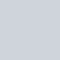# 前言

iou 全名為 intersection over union，

# iou 的概念與公式

iou 基本上 = 兩矩形的交集 / 兩矩形的聯集

• 完全重合時：得到最大值 1
• 完全不重合時：得到最小值 0
• 部分重合：得到 0~1 範圍的值

## 以下為圖解• 圖片引用自：https://blog.csdn.net/IAMoldpan/article/details/78799857

# 用 C++ 實作計算 iou 的 function – 網路常見「錯誤」示範

## iou – 常見「錯誤」範例程式碼

``````#include <iostream>
#include <opencv2/core/core.hpp>
#include <opencv2/highgui/highgui.hpp>

using namespace cv;
using namespace std;

int main()
{
Rect bbox_1(10, 20, 30, 40);
Rect bbox_2(20, 30, 40, 50);

Rect bbox_and;
bbox_and = bbox_1 & bbox_2;

Rect bbox_or;
bbox_or = bbox_1 | bbox_2;

cout<< "bbox_and.area() = " << bbox_and.area() << endl;
cout<< "bbox_or.area() = " << bbox_or.area() << endl;

cout<< "iou = " << (bbox_and.area()*1.0 / bbox_or.area()) << endl;

return 0;
}
``````

## 編譯與結果

``````> g++ iou.cpp -o iou.out -std=c++11 `pkg-config --cflags opencv`; ./iou.out

bbox_and.area() = 600
bbox_or.area() = 3000
iou = 0.2
``````

# 「正確」實驗結果分析• 首先是紅框 (bbox_1)，面積為 30*40=1200
• 再來是綠框 (bbox_2)，面積為 40*50=2000
• 紅綠框交疊部分 ，面積為 (40-20)*(60-30)=600
• 紅綠框聯集，面積為 1200+2000-600 = 2600 (兩個相加後，扣掉重複部分)

# 所以上面的程式問題出在哪?「Rectangle OR」算出的「藍色的部份 Rectangle」，

# 用 C++ 實作計算 iou 的 function – 「正確」示範

## iou – 「正確」範例程式碼

``````#include <iostream>
#include <opencv2/core/core.hpp>
#include <opencv2/highgui/highgui.hpp>

using namespace cv;
using namespace std;

int main()
{
Rect bbox_1(10, 20, 30, 40);
Rect bbox_2(20, 30, 40, 50);

Rect bbox_and;
bbox_and = bbox_1 & bbox_2;

float bbox_or = bbox_1.area() + bbox_2.area() - bbox_and.area();

cout<< "bbox_and.area() = " << bbox_and.area() << endl;
cout<< "bbox_or.area() = " << bbox_or << endl;

cout<< "iou = " << (bbox_and.area()*1.0 / bbox_or) << endl;

return 0;
}
``````

## 編譯與結果

``````> g++ iou.cpp -o iou.out -std=c++11 `pkg-config --cflags opencv`; ./iou.out

bbox_and.area() = 600
bbox_or.area() = 2600
iou = 0.230769
``````

# 想看 python 計算 iou 方法，可見我的另外一篇文

【OpenCV】用 python OpenCV 計算 iou 的方法 (內附範例程式碼) sample code

# Reference

⭐C++ 基礎用法 相關文章整理⭐：
1.【C++】C++ compile 程式碼 使用 c++ 11 與使用相關的 package
2.【C++】C/C++ 顯示資料的類別 (type) sample code (內含範例程式碼) print C data type, cout C++ data type, get variable type in c++
3.【C++】C++ 複製 2D array的方法 copy 2d array memcpy sample code (內含範例程式碼)
⭐C++ 字串處理相關文章整理⭐：
1.【C++】字串 char string stringstream 相關用法總整理 (內含範例程式碼) 與利用 sprinf, snprinf assign 值的方法
2.【C++】字串 char string stringstream 「轉換」用法總整理 (內含範例程式碼)
3.【C】printf, fprintf, sprintf, snprintf 相關用法總整理 (內含範例程式碼)
4.【C++】C++ String 用法 連接兩個 String c++ string concat
⭐C++ 系統偵測相關文章整理⭐：
1.【C++】C++ 利用 dirent.h 計算資料夾的檔案數量 count files sample code (內含範例程式碼)
2.【C++】C++ inotify sample code 偵測指定路徑底下的文件變化 (內有範例程式碼)
⭐C++ OpenCV 相關文章整理⭐：
1.【OpenCV】c++ OpenCV – 在 ubuntu 上第一次執行 OpenCV 程式 sample code (內含範例程式碼)
2.【OpenCV】c++ OpenCV - 在圖片上寫上文字 cv::putText sample code (內含範例程式碼)
3.【OpenCV】c++ OpenCV - cv::Rect 矩形用法與相關功能函數 sample code (內含範例程式碼)
4.【OpenCV】c++ OpenCV - OpenCV 中的純量 定義顏色 cv::Scalar(255,255,255) color sample code (內含範例程式碼)
5.【OpenCV】用 C++ 計算 iou 的方法 與網路算法常見錯誤(內附範例程式碼) sample code
⭐【喜歡我的文章嗎? 歡迎幫我按讚~ 讓基金會請創作者喝一杯咖啡!### 1 則留言

1. #### 【OpenCV】用 python OpenCV 計算 iou 的方法 (內附範例程式碼) sample code

[…] 【OpenCV】用 C++ 計算 iou 的方法 與網路算法常見錯誤(內附範例程式碼) sample c… […]